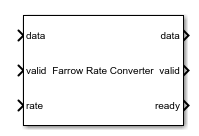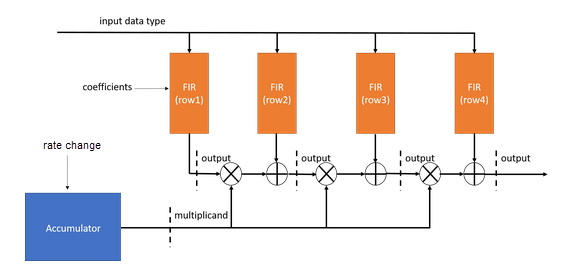# Farrow Rate Converter

Polynomial sample-rate converter

Since R2022a

•Libraries:
DSP HDL Toolbox / Signal Operations

## Description

The Farrow Rate Converter block converts the sample rate of a signal by using FIR filters to implement a polynomial sinc approximation. A Farrow filter is an efficient rate converter when the rate conversion factor is a ratio of large integer decimation and interpolation factors. Specify the rate conversion factor by providing the input sample rate and the desired output sample rate. You can provide the rate conversion factor as a fixed parameter or as a time-varying input signal.

You can use this block with the default coefficients for most rate conversions. The default coefficients are a LaGrange interpolation that matches the Farrow Rate Converter block in DSP System Toolbox™. Or, you can specify a custom set of coefficients if the default does not meet your specifications.

The block provides a hardware-friendly interface with input and output control signals. To provide a cycle-accurate simulation of the generated HDL code, the block models architectural latency including pipeline registers and multiplier optimizations.

## Ports

### Input

expand all

Input data, specified as a real or complex scalar. When the input data type is an integer type or a fixed-point type, the block uses fixed-point arithmetic for internal calculations.

The software supports `double` and `single` data types for simulation, but not for HDL code generation.

Data Types: `fixed point` | `single` | `double` | `int8` | `int16` | `int32` | `uint8` | `uint16` | `uint32`
Complex Number Support: Yes

Control signal that indicates if the input data is valid. When valid is `1` (`true`), the block captures the values from the input data port. When valid is `0` (`false`), the block ignores the values from the input data port.

Data Types: `Boolean`

Specify the rate change factor as a positive rational value that is the ratio of the input sample rate and the output sample rate, `Fin/Fout`. There are no limits on the rate change factor.

When this input value changes, the block resets the internal phase accumulator. This reset means you can change the rate change factor from decimation to interpolation. For example, you can use this block to align data streams that have similar but varying sample clocks.

The block derives the data type of the internal accumulator from the data type of this signal. The data type of the rate change must have at least one integer bit and one fractional bit. The accumulator data type is `fixdt(1,fractionalWL+1,fractionalWL)`, where fractionalWL is the fraction length of the rate change data type. The fractionalWL determines the accuracy of the phase timing, but also increases the critical path. When the rate change word length is large, you can limit hardware resource use by fitting the multiplicand data type to the DSP blocks on the FPGA. .

#### Dependencies

To enable this port, set the Rate change source parameter to ```Input port```.

Data Types: `fixed point`

Control signal that clears internal states. When reset is `1` (`true`), the block stops the current calculation and clears internal states. When the reset is `0` (`false`) and the input valid is `1` (`true`), the block captures data for processing.

For more reset considerations, see the Reset Signal section on the Hardware Control Signals page.

#### Dependencies

To enable this port, on the Control Ports tab, select Enable reset input port.

Data Types: `Boolean`

### Output

expand all

Filtered output data, returned as a real or complex scalar. When the input data type is a floating-point data type, the output data inherits the data type of the input data. When the input data type is an integer type or a fixed-point type, the Output parameter on the Data Types tab controls the output data type.

Data Types: `fixed point` | `single` | `double`
Complex Number Support: Yes

Control signal that indicates if the data from the output data port is valid. When valid is `1` (`true`), the block returns valid data from the output data port. When valid is `0` (`false`), the values from the output data port are not valid.

Data Types: `Boolean`

Control signal that indicates that the block is ready for new input data sample on the next cycle. When ready is `1` (`true`), you can specify the data and valid inputs for the next time step. When ready is `0` (`false`), the block ignores any input data in the next time step.

Data Types: `Boolean`

## Parameters

expand all

### Main

You can enter a constant rate change as a parameter or provide a time-varying rate change by using an input port.

Selecting `Input port` enables the rate port on the block.

Specify the rate change factor as a ratio of the input sample rate and the output sample rate, `Fin/Fout`, or provide a positive rational value. There are no limits on the rate change factor. Specify the data type for this value by using the RateChange parameter on the Data Types tab.

#### Dependencies

To enable this parameter, set Rate change source to `Property`.

Data Types: `double`

Specify FIR filter coefficients as an M-by-N matrix of real values, where N is the number of filters and M is the number of coefficients in each filter. N must be less than six. The block implements a polynomial of order N – 1. The default value is a special closed-form LaGrange solution that accomplishes most rate changes.

Data Types: `double`

This block implements the FIR filter stages by using the same architectures as the Discrete FIR Filter block. Specify the HDL filter architecture as one of these structures:

• ```Direct form systolic``` — This architecture provides a fully parallel filter implementation that makes efficient use of Intel® and Xilinx® DSP blocks. For architecture details, see Fully Parallel Systolic Architecture.

• ```Direct form transposed``` — This architecture is a fully parallel implementation that is suitable for FPGA and ASIC applications. For architecture and performance details, see Fully Parallel Transposed Architecture.

All implementations share multipliers for symmetric and antisymmetric coefficients and remove multipliers for zero-valued coefficients.

Serialization requirement for input timing, specified as a positive integer. This parameter represents N, the minimum number of cycles between valid input samples. To implement a fully serial architecture, set Minimum number of cycles between valid input samples greater than the filter length, L, or to `Inf`.

When this parameter is greater than one, the block implements each FIR subfilter as a partly-serial architecture that shares the multipliers in time.

#### Dependencies

To enable this parameter, set Filter structure to ```Direct form systolic```.

### Data Types

Rounding mode for type-casting the output to the data type specified by the Output parameter. When the input data type is a floating-point data type, the block ignores this parameter. For more details, see Rounding Modes.

Overflow handling for type-casting the output to the data type specified by the Output parameter. When the input data type is a floating-point data type, the block ignores this parameter. For more details, see Overflow Handling.

The block casts the filter coefficients to this data type. The quantization rounds to the nearest representable value and saturates on overflow. When the input data type is a floating-point data type, the block ignores this parameter.

The recommended data type for this parameter is ```Inherit: Same word length as input```. When selecting this data type, consider the size supported by the DSP blocks on your target FPGA.

The block casts the Rate change (fsin/fsout) parameter value to this data type and uses this data type to derive the data type for the internal accumulator. The accumulator data type is `fixdt(1,fractionalWL+1,fractionalWL)`, where fractionalWL is the fraction length of the rate change data type. The quantization rounds to the nearest representable value and saturates on overflow. When the input data type is a floating-point data type, the block ignores this parameter.

This data type must have enough integer bits to represent the `fsIn/fsOut` value. If the data type specified does not have enough integer bits, the block returns an error. The default setting does not specify a number of fractional bits, so the block can compute the necessary integer bits. This data type must have at least one integer bit and one fractional bit. The fractional part of this data type determines the accuracy of the phase timing, but also increases the critical path. When the rate change word length is large, you can limit hardware resources by fitting the multiplicand data type to the DSP blocks on the FPGA.

#### Dependencies

To enable this parameter, set Rate change source to `Property`.

The block casts the output of the accumulator to this data type. The quantization rounds to the nearest representable value and saturates on overflow. When the input data type is a floating-point data type, the block ignores this parameter. When the rate change word length is large, you can limit hardware resource use by controlling the multiplicand data type. When selecting this data type, consider the size supported by the DSP blocks on your target FPGA.

The block casts the output of each filter stage to this data type. The quantization uses the settings of the Rounding mode and Overflow mode parameters. When the input data type is a floating-point data type, the block ignores this parameter.

### Control Ports

Select this check box to enable the reset input port. The reset signal implements a local synchronous reset of the data path registers.

For more reset considerations, see the Reset Signal section on the Hardware Control Signals page.

Select this check box to connect the generated HDL global reset signal to the data path registers. This parameter does not change the appearance of the block or modify simulation behavior in Simulink®. When you clear this check box, the generated HDL global reset clears only the control path registers. The generated HDL global reset can be synchronous or asynchronous depending on the HDL Code Generation > Global Settings > Reset type parameter in the model Configuration Parameters.

For more reset considerations, see the Reset Signal section on the Hardware Control Signals page.

## Algorithms

expand all

The Farrow Rate Converter block uses Horner’s rule to compute samples from the polynomial. The polynomial is implemented with several FIR filters. Each FIR filter is an instance of the Discrete FIR Filter block. For details of filter architectures and resource optimization, see FIR Filter Architectures for FPGAs and ASICs. When Minimum number of cycles between valid input samples is greater than one, the block implements each FIR subfilter as a partly-serial architecture that shares the multipliers in time.

This diagram shows the Farrow architecture for a four-stage filter. There are four FIR filters. The output of each filter is added to the result of the previous stage, cast to the output data type, then multiplied by the current value of the accumulator. The accumulator operates on the fractional part of the rate change, and uses a data type derived from the rate change data type. The accumulator data type is `fixdt(1,fractionalWL+1,fractionalWL)`, where fractionalWL is the fraction length of the rate change data type.When you set the coefficient data type to ```Same as input wordlength```, each subfilter computes the best precision data type based on its coefficients. As a result, each filter can have a different output data type. To maintain full precision when you set the output data type to `Full precision`, the block casts the output of each subfilter to the highest precision data type of all the subfilter data types.

The table shows the coefficient data type and output data type for each subfilter when using the default Farrow coefficients, and an input data type of `fixdt(1,18,14)`.

Coefficient ValueCoefficient Data TypeSubfilter Output Data Type
`-1/6 1/2 -1/2 1/6``fixdt(1,18,17)``fixdt(1,36,31)`
`1/2 -1 1/2 0``fixdt(1,18,17)``fixdt(1,36,31)`
`-1/3 -1/2 1 -1/6``fixdt(1,18,16)``fixdt(1,35,30)`
`0 1 0 0``fixdt(1,18,16)``fixdt(1,34,30)`

In this case, the full precision data type is `fixdt(1,36,31)`.

## Version History

Introduced in R2022a

expand all